Graphing Sine, Cosine, and Tangent
 Home > Lessons > Graphing Sin, Cos, and Tan Search | Updated June 29th, 2023
Introduction
In this webpage, you will learn how to graph sine, cosine, and tangent functions. Here are the sections within this webpage:

 Basics The graphs of trigonometric functions have several properties to elicit. To be able to graph these functions by hand, we have to understand them. Before we progress, take a look at this video that describes some of the basics of sine and cosine curves.ideo: Graphing Basics: Sine and Cosine Terminology The video in the previous section described several parameters. This section will define them with precision within the following table.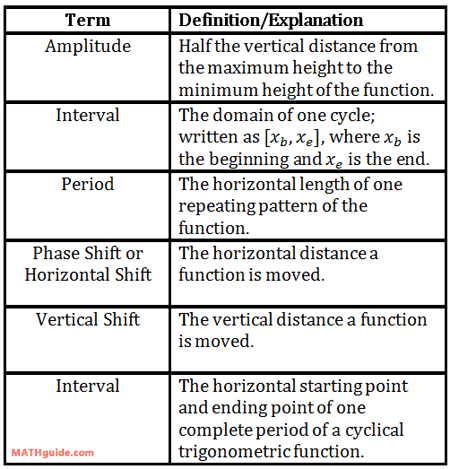Calculations Amplitude and Period To calculate amplitude and period, the equation of our sine and cosine curves have to be in a specific form. The equations have to look like this.Once in that form, the parameters for amplitude and period are calculated as follows.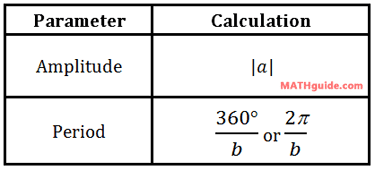Graphing Sine Amplitude and Period Here is a sine function we will graph.The a-value is the number in front of the sine function, which is 2. This makes the amplitude equal to |2| or simply 2. The graph of the function has a maximum y-value of 2 and a minimum y-value of -2.     The b-value is the number next to the x-term, which is 3. This means the period is 360 degrees divided by 3 or 120. So, the curve has a y-intercept of zero (because it is a sine curve it passes through the origin) and it completes one cycle in 120 degrees.     This is the graph of the sine curve.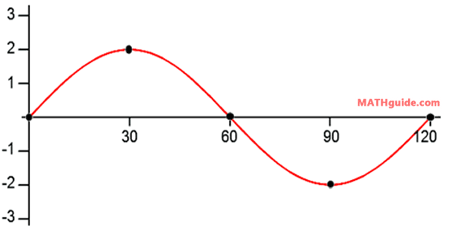This particular interval of the curve is obtained by looking at the starting point (0,0) and the end point (120,0). The domain (the x-values) of this cycle go from 0 to 120. So, we write this interval as [0,120].     This video will demonstrate how to graph a different sine function with two parameters: amplitude and period.ideo: Graphing Sine: Amplitude and Perioduiz: Graphing Sine & Cosine: Amplitude and Period Graphing Cosine Amplitude and Period Here is a cosine function we will graph.The a-value is the number in front of the sine function, which is 4. This makes the amplitude equal to |4| or 4. The graph of the function has a maximum y-value of 4 and a minimum y-value of -4.     The b-value is the number next to the x-term, which is 2. This means the period is 360 degrees divided by 2 or 180. So, the curve has a y-intercept at its maximum (0,4) (because it is a cosine curve) and it completes one cycle in 180 degrees.     This is the graph of the cosine curve.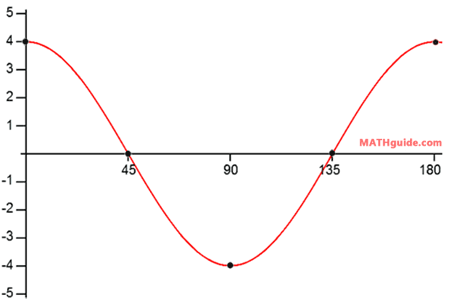This particular interval of the curve is obtained by looking at the starting point (0,4) and the end point (180,4). The domain (the x-values) of this cycle go from 0 to 180. So, we write this interval as [0,180].     This video will demonstrate how to graph a different cosine function with two parameters: amplitude and period.ideo: Graphing Cosine: Amplitude and Perioduiz: Graphing Sine & Cosine: Amplitude and Period Calculations Phase Shift and Vertical Shift To calculate phase shift and vertical shift, the equation of our sine and cosine curves have to be in a specific form. The equations have to look like this.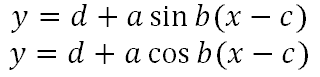Once in that form, all the parameters can be calculated as follows.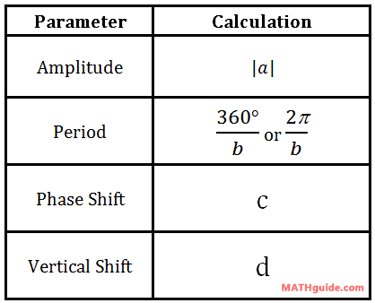Notice that the equations have subtraction signs inside the parentheses. The c-values have subtraction signs in front of them. However, the phase shift is the opposite. This will be demonstrated in the next two sections. Graphing Sine Amp, Period, Phase Shift, and Vert. Shift This video will demonstrate how to graph a sine function with four parameters: amplitude, period, phase shift, and vertical shift.ideo: Graphing Sine: Amplitude, Period, Phase Shift, and Vertical Shiftuiz: Graphing Sine/Cosine: Amplitude, Period, Phase Shift, and Vertical Shift Graphing Cosine Amp, Period, Phase Shift, and Vert. Shift This video will demonstrate how to graph a cosine function with four parameters: amplitude, period, phase shift, and vertical shift.ideo: Graphing Cosine: Amplitude, Period, Phase Shift, and Vertical Shiftuiz: Graphing Sine/Cosine: Amplitude, Period, Phase Shift, and Vertical Shift Tide Sinusoid Problem Word Problem The ocean level rises and falls. It is referred to as the tide. This video will explain how to use a set of facts that are laid out in a word problem and create the equation of a sinusoid. The equation will quantify the height of the tide over time.ideo: Tide Sinusoid Problem Graphing Tangent Period and Phase Shift This video will demonstrate how to graph a tangent function with two parameters: period and phase shift.ideo: Graphing Tangent: Period and Phase Shift Videos Try our instructional videos on the lessons above.ideo: Graphing Basics: Sine and Cosineideo: Graphing Sine: Amplitude and Periodideo: Graphing Cosine: Amplitude and Periodideo: Graphing Sine: Amplitude, Period, Phase Shift, and Vertical Shiftideo: Graphing Cosine: Amplitude, Period, Phase Shift, and Vertical Shiftideo: Tide Sinusoid Problemideo: Graphing Tangent: Period and Phase Shiftideo: The Unit Circle: Trigonometry Quizmasters Here is an interative quiz.uiz: Graphing Sine & Cosine: Amplitude and Perioduiz: Graphing Sine/Cosine: Amplitude, Period, Phase Shift, and Vertical Shiftuiz: Graphs to Equationsuiz: Tide STEM Problem Activities Here are activities replated to the lessons in this section.ctivity: Graphing Trig Functions [amplitude, period]ctivity: Graphing Trig Functions 2 [amplitude, period, phase shift, horizontal shift]ctivity: Graphs to Equations [amplitude, period, interval, y-intercept, equation]ctivity: Tide Problem: Preliminaryctivity: Tide Problem: CCSS.MATH.CONTENT.HSF.TF.B.5ctivity: Tide STEM Problem Related Lessons Try this advanced lesson, which is closely related to the lessons above.esson: Equations of Musical Notesesson: Graphing Linesesson: Graphing Linear Inequalitiesesson: Graphing Conic Sectionsesson: Linear Programming